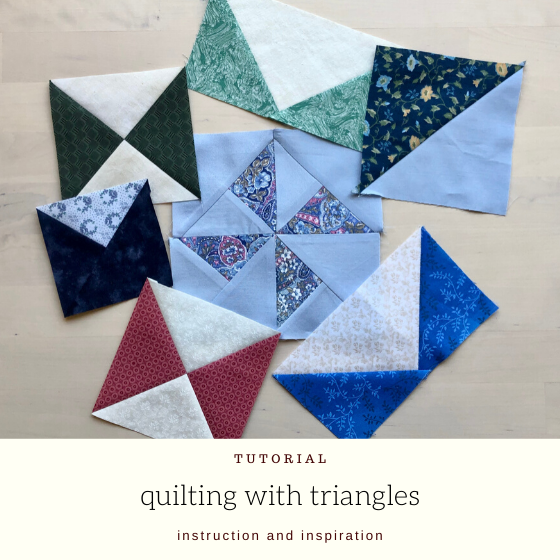# Quilting with Triangles

## Half Square Triangles

Half square triangles are triangles that are made by cutting a square on the diagonal. The term refers to both the triangles and the squares that are made by stitching two half square triangles together.

They are also commonly called “HST’s”.

There are many different methods to making half square triangles. Every quilter has their favourite. Try a few different ones and you will find one that you like.

## Flying Geese

Flying geese are made with a rectangle of fabric and a triangle on either side. You can make these by joining two half square triangles, but that leaves a seam in the middle. Making them as a single unit reduces bulk and makes quilting easier.

## Quarter Square Triangles

Quarter square triangles are made by cutting squares into four on the diagonals. They can be stitched back together into units with two or four different fabrics.

## Split Half-square Triangles

Split half-square triangles are a combination of half square triangles and quarter square triangles. They can be made with two or three fabrics for different effects.

### Common methods for construction:

This method is intuitive – nothing to think about.

Paper piecing means perfectly accurate results.

This popular method makes four at a time.

## Quilts with Triangles

Here are some quilts made with triangles for inspiration.## Elizabeth DeCroos

designer, teacher, speaker